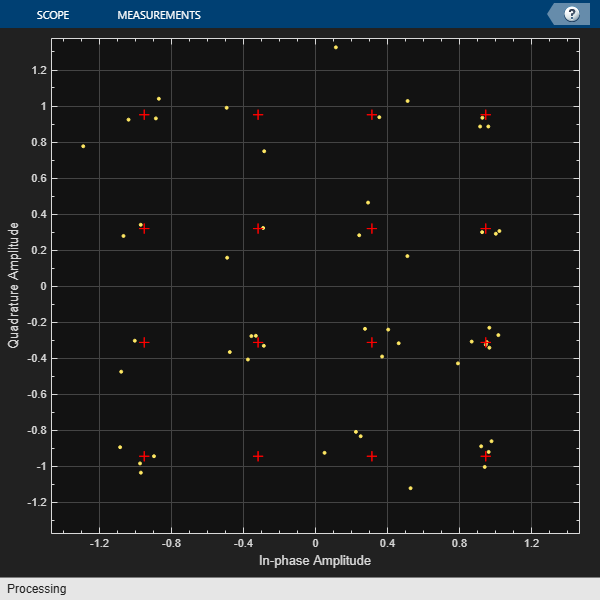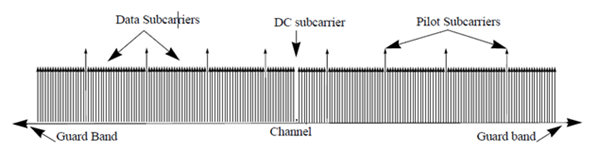# ofdmdemod

Demodulate using OFDM method

## Syntax

``Y = ofdmdemod(X,nfft,cplen)``
``Y = ofdmdemod(X,nfft,cplen,symOffset)``
``Y = ofdmdemod(X,nfft,cplen,symOffset,nullidx)``
``[Y,pilots] = ofdmdemod(X,nfft,cplen,symOffset,nullidx,pilotidx)``
``Y = ofdmdemod(X,nfft,cplen,___,OversamplingFactor=Value)``

## Description

example

````Y = ofdmdemod(X,nfft,cplen)` demodulates the input time domain signal specified in `X` using the orthogonal frequency division multiplexing (OFDM) method with an FFT size specified by `nfft` and cyclic prefix length specified by `cplen`. For information, see OFDM Demodulation.```
````Y = ofdmdemod(X,nfft,cplen,symOffset)` applies the symbol sampling offset, `symOffset`, for each OFDM symbol before demodulation of the input.```

example

````Y = ofdmdemod(X,nfft,cplen,symOffset,nullidx)` removes null subcarriers from the locations specified in `nullidx`. For this syntax, the symbol sampling offset is applied to each OFDM symbol and the number of rows in the output is `nfft` – `length(nullidx)`, which accounts for the removal of null subcarriers. Use null subcarriers to account for guard bands and DC subcarriers. For information, see Subcarrier Allocation and Guard Bands.```

example

````[Y,pilots] = ofdmdemod(X,nfft,cplen,symOffset,nullidx,pilotidx)` returns pilot subcarriers for the pilot indices specified in `pilotidx`. For this syntax, the symbol sampling offset is applied to each OFDM symbol and number of rows in the output `Y` is `nfft` – `length(nullidx)` – `length(pilotidx)`, which accounts for the removal of null and pilot subcarriers. The function assumes that pilot subcarrier locations are the same across each OFDM symbol and transmit antenna.```

example

````Y = ofdmdemod(X,nfft,cplen,___,OversamplingFactor=Value)` specifies the optional oversampling factor name-value argument in addition to input arguments in previous syntaxes. The oversampling factor for an upsampled input signal must be specified as a positive scalar, and the products (`OversamplingFactor`×`nfft`) and (`OversamplingFactor`×`cplen`) must both result in integers. The default value for `OversamplingFactor` is `1`.For example, `ofdmdemod(inSym,nfft,cplen,OversamplingFactor=2)` demodulates assuming the input signal was upsampled by a factor of two. TipIf you set the oversampling factor to an irrational number, specify the fractional value. For example, with an FFT length of `12` and an oversampling factor of `4/3`, their product is the integer `16`. However, rounding `4/3` to `1.333` when setting the oversampling factor results in a noninteger product of `15.9960`, which results in a code error. ```

## Examples

collapse all

OFDM-demodulate a signal with different CP lengths for different symbols.

Initialize input parameters defining locations for null and pilot subcarriers. Generate random data and perform OFDM modulation.

```M = 16; nfft = 64; cplen = [16 32]; nSym = 2; dataSym = randi([0 M-1],nfft,nSym); qamSig = qammod(dataSym,M,UnitAveragePower=true); y1 = ofdmmod(qamSig,nfft,cplen);```

Demodulate the OFDM symbols. Compare the results to the original input data. The difference between the signals is negligible.

```x1 = ofdmdemod(y1,nfft,cplen); rxData = qamdemod(x1,M,UnitAveragePower=true); isequal(rxData,dataSym)```
```ans = logical 1 ```

Apply OFDM multiplexing to a 16-QAM signal filtered by a SISO link with Rayleigh fading.

Initialize simulation variables, and create Rayleigh fading channel and constellation diagram objects.

```s1 = RandStream('mt19937ar',Seed=12345); nFFT = 64; cpLen = 16; nullIdx = [1:6 33 64-4:64].'; numTones = nFFT-length(nullIdx); k = 4; % bits per symbol M = 2^k; constSym = qammod((0:M-1),M, ... UnitAveragePower=true); % reference constellation symbols maxDopp = 1; pathDelays = [0 4e-3 8e-3]; pathGains = [0 -2 -3]; sRate = 1000; sampIdx = round(pathDelays/(1/sRate)) + 1; chan = comm.RayleighChannel(PathGainsOutputPort=true, ... MaximumDopplerShift=maxDopp, ... PathDelays=pathDelays, ... AveragePathGains=pathGains, ... SampleRate=sRate, ... RandomStream='mt19937ar with seed'); cdScope = comm.ConstellationDiagram( ... ShowReferenceConstellation=true, ... ReferenceConstellation=constSym);```

Generate signal data and apply 16-QAM modulation.

```data = randi(s1,[0 M-1],numTones,1); modOut = qammod(data,M,UnitAveragePower=true);```

Apply OFDM modulation and pass the signal through the channel.

```y = ofdmmod(modOut,nFFT,cpLen,nullIdx); [fadSig,pg] = chan(y);```

Determine symbol sampling offset.

`symOffset = min(max(sampIdx),cpLen)`
```symOffset = 9 ```

OFDM demodulate the received signal with a time shift. Display the constellation diagram before equalization.

```x = ofdmdemod(fadSig,nFFT,cpLen,symOffset,nullIdx); cdScope(x);```Convert the vector of path gains, `pg`, to scalar tap gains that correspond to data subcarriers, `h_datasubcarr`. Use the `h_datasubcarr` tap gains for equalization during signal recovery.

```hImp = complex(zeros(nFFT,1)); hImp(sampIdx) = mean(pg,1); hall = fftshift(fft(hImp)); dataIdx = setdiff((1:nFFT)',nullIdx); h_datasubcarr = hall(dataIdx);```

Equalize the signal. Display the constellation diagram after equalization.

```eqSig = x ./ h_datasubcarr; cdScope(eqSig);```Demodulate the 16-QAM symbols to recover the signal. Compute the symbol error rate.

```rxSym = qamdemod(eqSig,M,UnitAveragePower=true); numErr = symerr(data,rxSym); disp(['Number of symbol errors: ' num2str(numErr) ... ' out of ' num2str(length(data)) ' symbols.'])```
```Number of symbol errors: 2 out of 52 symbols. ```

OFDM-demodulate data input that includes null and pilot packing.

Initialize input parameters, defining locations for null and pilot subcarriers. Generate random data and perform OFDM modulation.

```Mqam = 16; Mpsk = 4; nfft = 64; cplen = 16; nSym = 10; nullIdx = [1:6 33 64-4:64]'; pilotIdx = [12 26 40 54]'; numDataCarrs = nfft-length(nullIdx)-length(pilotIdx); dataSym = randi([0 Mqam-1],numDataCarrs,nSym); qamSig = qammod(dataSym,Mqam,UnitAveragePower=true); pilotSym = repmat((0:Mpsk-1).',1,nSym); pilots = pskmod(pilotSym,Mpsk); y2 = ofdmmod(qamSig,nfft,cplen,nullIdx,pilotIdx,pilots);```

Demodulate the OFDM symbols. Compare the results to the original input data to show that the demodulated signal and the original data and pilot signals are equal.

```symOffset = cplen; [x2,rxPilots] = ofdmdemod(y2,nfft,cplen,symOffset,nullIdx,pilotIdx); rxData = qamdemod(x2,Mqam,UnitAveragePower=true); isequal(rxData,dataSym)```
```ans = logical 1 ```
```rxPilotSym = pskdemod(rxPilots,Mpsk); isequal(rxPilotSym,repmat((0:Mpsk-1).',1,nSym))```
```ans = logical 1 ```

Demodulate an oversampled OFDM modulation that has a sample offset. Insert nulls in the OFDM grid and oversample the output signal.

Initialize variables for the oversampling factor, FFT size, cyclic prefix length, and sample offset.

```M = 64; osf = 4/3; nfft = 768; cplen = 24; sampOffset = 5; symOffset = cplen - (sampOffset/osf);```

Generate data symbols and OFDM-modulate the data.

```dataSym = randi([0 M-1],nfft,1); qamSig = qammod(dataSym,M,UnitAveragePower=true); y3 = ofdmmod(qamSig,nfft,cplen,OversamplingFactor=osf);```

Demodulate the signal and show the demodulated data symbols match the original input data symbols.

```x3 = ofdmdemod(y3,nfft,cplen,symOffset,OversamplingFactor=osf); rxSym = qamdemod(x3,M,UnitAveragePower=true); isequal(rxSym,dataSym)```
```ans = logical 1 ```

## Input Arguments

collapse all

Modulated OFDM symbols, specified as a NS-by-NR numeric array or `dlarray` (Deep Learning Toolbox) object.

• If `cplen` is a scalar, the number of samples, NS, equals (`nfft` + `cplen`) × NSym

• If `cplen` is a row vector, NS equals (`nfft` × NSym) + `sum`(`cplen`)

NSym is the number of symbols per antenna and NR is the number of receive antennas.

• For deep learning workflows, `X` can be an array of up to three dimensions, specified as NS-by-NR-by-Nb array. Nb is the number of batch observations in the deep learning workflow.

Data Types: `double` | `single`
Complex Number Support: Yes

FFT length, specified as an integer greater than or equal to 8. `nfft` is equivalent to the number of subcarriers used in the demodulation process.

Data Types: `double`

Cyclic prefix length, specified as a scalar or as a row vector of length NSym.

• When you specify `cplen` as a scalar, the cyclic prefix length is the same for all symbols through all antennas.

• When you specify `cplen` as a row vector of length NSym, the cyclic prefix length can vary across symbols but remains the same length through all antennas.

Data Types: `double`

Symbol sampling offset, specified as values from 0 to `cplen`.

• If you do not specify `symOffset`, the default value is an offset equal to `cplen`.

• If you specify `symOffset` as a scalar, the same offset is used for all symbols.

• If you specify `symOffset` as a row vector, the offset value can be different for each symbol.

For information, see Windowing and Symbol Offset.

Data Types: `double`

Indices of null subcarrier locations, specified as a column vector with element values from 1 to `nfft`. If you specify `nullidx`, the number of rows in `Y` is `(nfft-length(nullidx))`. For information, see Subcarrier Allocation and Guard Bands.

Data Types: `double`

Indices of pilot subcarrier locations, specified as a column vector with element values from 1 to `nfft`. If you specify `pilotidx`, the number of rows in `Y` is (`nfft` – length(`nullidx`) – length(`pilotidx`)).For information, see Subcarrier Allocation and Guard Bands.

Data Types: `double`

## Output Arguments

collapse all

Output demodulated symbols, returned as an NData-by-NSym-by-NR array of symbols or `dlarray` (Deep Learning Toolbox) object. The output data format matches the input signal, `X`.

For information, see OFDM Demodulation.

Pilot subcarriers, returned as an NPilot-by-NSym-by-NR array of symbols or `dlarray` (Deep Learning Toolbox) object. The output data format matches the input signal, `X`.

• NPilot equals the length of `pilotidx`.

• NSym is the number of OFDM symbols per antenna.

• NR is the number of receive antennas.

The function assumes that the pilot subcarrier locations are the same across each OFDM symbol and transmit antenna. To vary pilot subcarrier locations across OFDM symbols or antennas, use the `comm.OFDMDemodulator` System object™.

## Algorithms

collapse all

### OFDM Demodulation

The orthogonal frequency division multiplexing (OFDM) method demodulates an OFDM input signal by using an FFT operation that results in N parallel data streams.

The figure shows an OFDM demodulator consisting of a bank of N correlators with one correlator assigned to each OFDM subcarrier. A parallel-to-serial conversion follows the correlator bank.### Subcarrier Allocation and Guard Bands

Individual OFDM subcarriers are allocated as data, pilot, or null subcarriers.

As shown here, subcarriers are designated as data, DC, pilot, or guard-band subcarriers.• Data subcarriers transmit user data.

• Pilot subcarriers are for channel estimation.

• Null subcarriers transmit no data. Subcarriers with no data provide a DC null and serve as buffers between OFDM resource blocks.

• The null DC subcarrier is the center of the frequency band with an index value of (`nfft`/2 + 1) if `nfft` is even, or ((`nfft` + 1) / 2) if `nfft` is odd.

• The guard bands provide buffers between adjacent signals in neighboring bands to reduce interference caused by spectral leakage.

Null subcarriers enable you to model guard bands and DC subcarrier locations for specific standards, such as the various 802.11 formats, LTE, WiMAX, or for custom allocations. You can allocate the location of nulls by assigning a vector of null subcarrier indices.

Similar to guard bands, guard intervals protect the integrity of transmitted signals in OFDM by reducing intersymbol interference.

Assignment of guard intervals is analogous to the assignment of guard bands. You can model guard intervals to provide temporal separation between OFDM symbols. The guard intervals help preserve intersymbol orthogonality after the signal passes through time-dispersive channels. You create guard intervals by using cyclic prefixes. Cyclic prefix insertion copies the last part of an OFDM symbol as the first part of the OFDM symbol.OFDM benefits from the use of cyclic prefix insertion as long as the span of the time dispersion does not exceed the duration of the cyclic prefix.

Inserting a cyclic prefix results in a fractional reduction of user data throughput because the cyclic prefix occupies bandwidth that could be used for data transmission.

### Windowing and Symbol Offset

To reduce intersymbol interference (ISI) introduced by signal windowing applied at the transmitter, a fractional symbol offset is applied before demodulation of each OFDM symbol. Signal windowing is often applied to transmitted OFDM symbols to smooth the discontinuity between consecutive OFDM symbols. Windowing reduces intersymbol out-of-band emissions but increases ISI.

The windowed OFDM symbol consists of the cyclic prefix (CP), ODFM symbol data, plus windowing regions at the beginning and end of the symbol. The leading and trailing windowing shoulders have tails as shown in this figure.To reduce ISI, you can align signal sample timing by specifying a symbol sampling offset that gets applied before OFDM symbol demodulation.Specify the symbol sampling offset as a value in the range [0, LCP], where LCP is the cyclic prefix length.

• When the symbol sampling offset is a scalar, the FFT window begins at the X+1 sample of the cyclic prefix.

• When the symbol sampling offset is zero, no offset is applied and the FFT window starts at the first sample of the symbol.

• When the symbol sampling offset is LCP, the FFT window begins after the last CP sample. If symbol sampling offset is not specified, LCP is the default setting.

## Version History

Introduced in R2018a

expand all